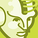# App Development

Announcements
QlikWorld online is next week! REGISTER NOW
cancel
Showing results for
Did you mean:Contributor II

## Calculated Dimension (?)

Currently, I have  =RegionCode as a 2nd dimension in a bar chart, where RegionCode is a table field.  The request is to put a sales variance count after the RegionCode.  So I'm trying to concatenate RegionCode with a set expression as follows, and keep getting "Invalid Dimension".

RegionCode & ' - ' &

(

count({< StatusCurr={'Actual'}, SourceCurr={'JDE'}, ActivityTypeCurr={'Agreement TBI'} , LotStatusCodeCurr={\$(vLotStatusCode)}, ActivityDateCurr={">=\$(=Timestamp(YearStart(left((vCurrentDate),10), 0,11)))<=\$(=Timestamp(left((vCurrentDate),10)))"}>} ActivityTypeCurr)
-
count({< StatusCurr={'Actual'}, SourceCurr={'JDE'}, ActivityTypeCurr={'Agreement Lost'} , LotStatusCodeCurr={\$(vLotStatusCode)}, ActivityDateCurr={">=\$(=Timestamp(YearStart(left((vCurrentDate),10), 0,11)))<=\$(=Timestamp(left((vCurrentDate),10)))"}>} ActivityTypeCurr)

)

0 RepliesTags